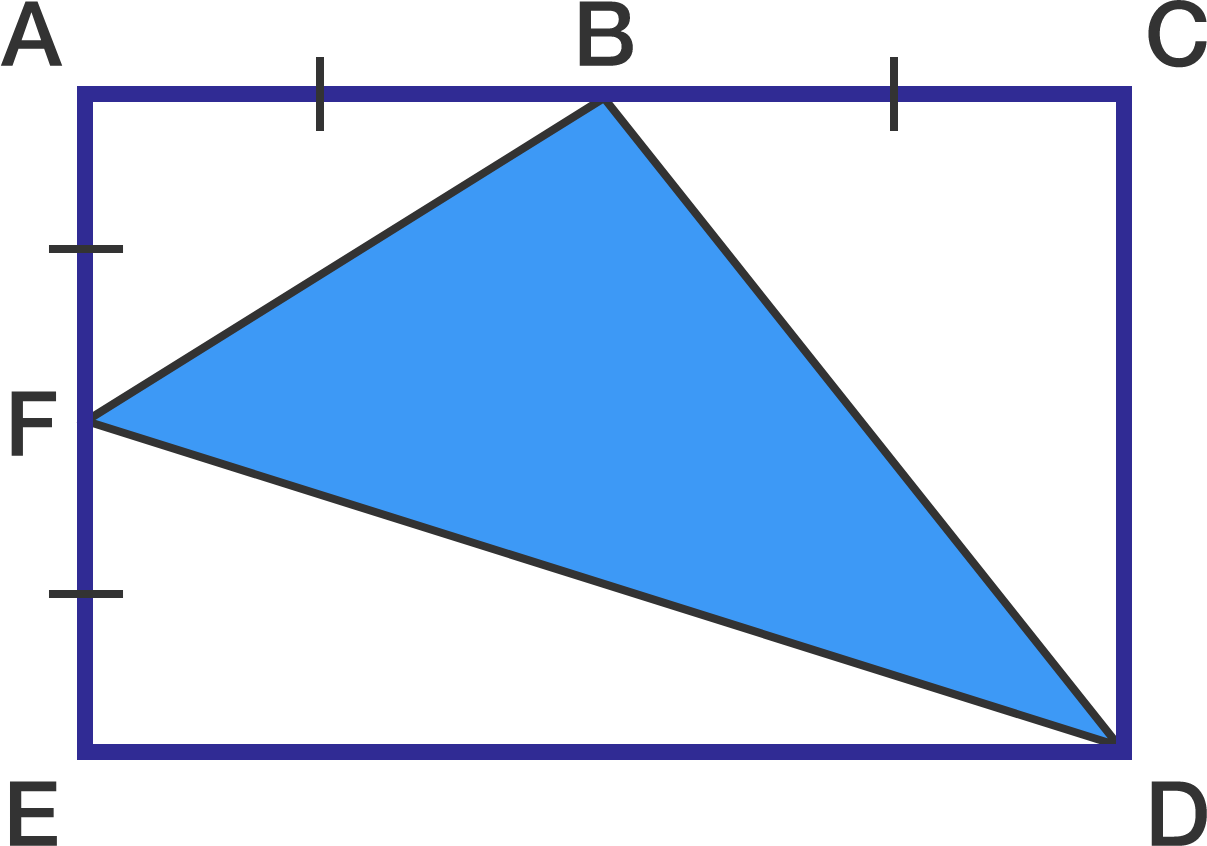# 3 - AreaThe rectangle in the diagram has an area equal to 640 $\text{cm}^2$.

Points B and F are midpoints of sides AC and AE, respectively.

What is the area of triangle BDF in $\text{cm}^2?$

×